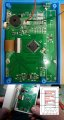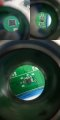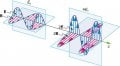# EMF/RF meter sensors on the pcb

#### xchcui

Joined May 12, 2014
257
Hi.
At the attached photo you may see the pcb of a simple EMF/RF meter. This meter shows,at the same time,the values of the electric field, magnetic field and power density.(see small illustration on the left/bottom of the photo).
Where are the sensors(magnetic field sensor,electric field sensor and RF radiation sensor)located in that circuit board?
Thanks.#### xchcui

Joined May 12, 2014
257

#### MisterBill2

Joined Jan 23, 2018
16,550
That PCB coil is another magnetic field pickup. It can also serve as an electric field sensor the same as a solid surface pickup plate.

•xchcui

#### xchcui

Joined May 12, 2014
257
That PCB coil is another magnetic field pickup. It can also serve as an electric field sensor the same as a solid surface pickup plate.
Are you refer to L2 coil?(red arrow No. 2 ?)
While you said"...another magnetic field pickup.."
where is the first magnetic field pickup besides that?
What does the spiral trace(red arrows No.1)sense?
Where is the RF radiation sensor?

#### MisterBill2

Joined Jan 23, 2018
16,550
The spiral trace is a magnetic pickup when it is connected as a coil, but with only one end connected it is a long antenna wire.

•xchcui

#### xchcui

Joined May 12, 2014
257
The spiral trace is a magnetic pickup when it is connected as a coil, but with only one end connected it is a long antenna wire.
I just figured out that PCB coil is actually refer to the spiral trace.
Okay,
1)The L2 coil may be the magnetic field sensor
2)The PCB coil(spiral trace)may be the electric field sensor,
( act the same as a solid surface pickup plate)
Where this third sensor located on the pcb?

#### xchcui

Joined May 12, 2014
257
Does Anyone have any idea where is the third sensor?
(The RF radiation sensor?)on that pcb?

#### MisterBill2

Joined Jan 23, 2018
16,550
Now I am wondering as to what the purpose of these questions is. Just curiosity? Or is there another reason?

#### xchcui

Joined May 12, 2014
257
Now I am wondering as to what the purpose of these questions is. Just curiosity? Or is there another reason?
It is a curiosity.I was curious to know,how does the emf/rf meter
that detects 3 types of energy do it.
I figured out two of them and i need to figure out where is
the third in order to complete the all picture.
I understood that:
1)the coil(L2)probably measures the magnetic field.
2)the PCB coil(spiral trace)probably detects the electric field.
(and yes,i saw on other emf meter that they are using solid
surface pickup plate,as you mentioned before)
But where is the sensor that detect the RF radiation
located on this PCB?

#### MisterBill2

Joined Jan 23, 2018
16,550
An inductance such as that same coil will pick up RF, the magnetic component, quite well. That is the principle of the loop antenna.

•xchcui

#### xchcui

Joined May 12, 2014
257
An inductance such as that same coil will pick up RF, the magnetic component, quite well. That is the principle of the loop antenna.
You are referring to the L2 coil,yes?(shape of loop antenna)
If yes,does it mean that only 2 sensor detects those 3 types of EM forces?
The electric field is detected by the PCB coil(spiral trace),while the magnetic field(uT,mG) and the electromagnetic RF radiation(uW/m2),them both,are detected by this L2 coil?

#### MisterBill2

Joined Jan 23, 2018
16,550
That is what I am thinking, yes.

#### nsaspook

Joined Aug 27, 2009
12,293
You are referring to the L2 coil,yes?(shape of loop antenna)
If yes,does it mean that only 2 sensor detects those 3 types of EM forces?
The electric field is detected by the PCB coil(spiral trace),while the magnetic field(uT,mG) and the electromagnetic RF radiation(uW/m2),them both,are detected by this L2 coil?
There is only one EM force. The two sensors detect the expression of that EM force as electric E (circuit theory voltage) and magnetic B (circuit theory current) component fields at the measurement points. With these two values you can compute the energy at that point using a normal power equation(s). Some of these equations assume a free space impedance value (permittivity/permeability of free space) so it's possible to do energy calculations with one EM component.

https://courses.lumenlearning.com/physics/chapter/24-4-energy-in-electromagnetic-waves/ note:Figure 1. Energy carried by a wave is proportional to its amplitude squared. With electromagnetic waves, larger E-fields and B-fields exert larger forces and can do more work.

note: The link talks about the resonant frequency of water molecules in the context of microwave ovens. Microwave ovens don't operate at the resonant frequency of water molecules.

https://www.schoolphysics.co.uk/age16-19/Wave properties/Wave properties/text/Microwave_ovens/index.html
Microwave ovens operate at a frequency of 2.45 GHz and this is NOT the resonant frequency of a water molecule. This frequency is much lower than the diatomic molecule resonant frequencies mentioned earlier. If 2.45 GHz were the resonant frequency of water molecules the microwaves would all be absorbed in the surface layer of a substance (liquid water or food) and so the interior of the food would not get cooked at all.

Last edited:
•xchcui

#### xchcui

Joined May 12, 2014
257
Okay,i think i got it.
If you have the value of the electric field and the value of the magnetic
field,the power density can be calculated,so 2 sensors are enough
for showing 3 parameters on the emf/rf meter.
Thanks alot for your help#### nsaspook

Joined Aug 27, 2009
12,293
Okay,i think i got it.
If you have the value of the electric field and the value of the magnetic
field,the power density can be calculated,so 2 sensors are enough
for showing 3 parameters on the emf/rf meter.
Thanks alot for your helpIt's easy once you understand the concept and useful to understand why some RF energy fears are unfounded.
1. Create Your Own Problem. Consider electromagnetic fields produced by high voltage power lines. Construct a problem in which you calculate the intensity of this electromagnetic radiation in W/m2 based on the measured magnetic field strength of the radiation in a home near the power lines. Assume these magnetic field strengths are known to average less than a μT. The intensity is small enough that it is difficult to imagine mechanisms for biological damage due to it. Discuss how much energy may be radiating from a section of power line several hundred meters long and compare this to the power likely to be carried by the lines. An idea of how much power this is can be obtained by calculating the approximate current responsible for μT fields at distances of tens of meters.

it takes some effort to,actually,understand the concept